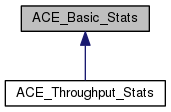ACE  6.2.8
ACE_Basic_Stats Class Reference

Collect basic stats about a series of samples. More...

`#include <Basic_Stats.h>`

Inheritance diagram for ACE_Basic_Stats:[legend]

## Public Types

typedef ACE_UINT64 scale_factor_type

## Public Member Functions

ACE_Basic_Stats (void)
Constructor. More...

ACE_UINT32 samples_count (void) const
The number of samples received so far. More...

void sample (ACE_UINT64 value)
Record one sample. More...

void accumulate (const ACE_Basic_Stats &rhs)
Update the values to reflect the stats in rhs. More...

void dump_results (const ACE_TCHAR *msg, scale_factor_type scale_factor) const
Dump all the samples. More...

## Public Attributes

ACE_UINT32 samples_count_
The number of samples. More...

ACE_UINT64 min_
The minimum value. More...

ACE_UINT32 min_at_
The number of the sample that had the minimum value. More...

ACE_UINT64 max_
The maximum value. More...

ACE_UINT32 max_at_
The number of the sample that had the maximum value. More...

ACE_UINT64 sum_
The sum of all the values. More...

## Detailed Description

Collect basic stats about a series of samples.

Compute the average and standard deviation (aka jitter) for an arbitrary number of samples, using constant space. Normally used for latency statistics.

## Constructor & Destructor Documentation

 ACE_Basic_Stats::ACE_Basic_Stats ( void )
inline

Constructor.

The number of samples is pre-allocated, and cannot changes once the class is initialized.

## Member Function Documentation

 void ACE_Basic_Stats::accumulate ( const ACE_Basic_Stats & rhs )

Update the values to reflect the stats in rhs.

 void ACE_Basic_Stats::dump_results ( const ACE_TCHAR * msg, ACE_Basic_Stats::scale_factor_type sf ) const

Dump all the samples.

Prints out the results, using msg as a prefix for each message and scaling all the numbers by scale_factor. The latter is useful because high resolution timer samples are acquired in clock ticks, but often presented in microseconds.

 void ACE_Basic_Stats::sample ( ACE_UINT64 value )
inline

Record one sample.

 ACE_UINT32 ACE_Basic_Stats::samples_count ( void ) const
inline

The number of samples received so far.

## Member Data Documentation

 ACE_UINT64 ACE_Basic_Stats::max_

The maximum value.

 ACE_UINT32 ACE_Basic_Stats::max_at_

The number of the sample that had the maximum value.

 ACE_UINT64 ACE_Basic_Stats::min_

The minimum value.

 ACE_UINT32 ACE_Basic_Stats::min_at_

The number of the sample that had the minimum value.

 ACE_UINT32 ACE_Basic_Stats::samples_count_

The number of samples.

 ACE_UINT64 ACE_Basic_Stats::sum_

The sum of all the values.

The documentation for this class was generated from the following files: